/
Features

# Action-Based Discretization for AI Search

As computer gaming reaches ever-greater heights in realism, we can expect the complexity of simulated dynamics to reach further as well. To populate such gaming environments with agents that behave intelligently, there must be some means of reasoning about the consequences of agent actions. Such ability to seek out the ramifications of various possible action sequences, commonly called “lookahead”, is found in programs that play chess, but there are special challenges that face game programmers who wish to apply AI search techniques to complex continuous dynamical systems. In particular, the game programmer must “discretize” the problem, that is, approximate the continuous problem as a discrete problem suitable for an AI search algorithm.

As computer gaming reaches ever-greater heights in realism, we can expect the complexity of simulated dynamics to reach further as well. To populate such gaming environments with agents that behave intelligently, there must be some means of reasoning about the consequences of agent actions. Such ability to seek out the ramifications of various possible action sequences, commonly called “lookahead”, is found in programs that play chess, but there are special challenges that face game programmers who wish to apply AI search techniques to complex continuous dynamical systems. In particular, the game programmer must “discretize” the problem, that is, approximate the continuous problem as a discrete problem suitable for an AI search algorithm.

As a concrete example, consider the problem of navigating a simulated submarine through a set of static obstacles. This continuous problem has infinite possible states (e.g. submarine position and velocity) and infinite possible trajectories. The standard approach to discretize the problem is to define a graph of “waypoints” between which the submarine can easily travel. A simple waypoint graph can be searched, but this approach is not without significant disadvantages.

First, the dynamics of such approximate navigation are not realistic. It’s still common to see massive vehicles in computer games turn about instantly and maintain constant velocity at all times. When considering acceleration in agent behavior, there’s a quick realization that the notion of a “waypoint” becomes far more complex. For example, a vehicle with realistic physical limitations cannot ignore momentum and turn a tight corner at any velocity. A generalized waypoint for such a system would contain not only a position vector, but a velocity vector as well, doubling the dimensions of the waypoint. If waypoint density is held constant, memory requirements grow exponentially with the waypoint dimensions.

The second disadvantage is that relevant state can incorporate many factors beyond waypoints in a dynamic environment. If the programmer wishes the submarine to pilot around moving obstacles, state dimensionality is further increased along with an exponential increase of the memory requirements for our state-based discretization.

An alternative way to look at the discretization of continuous search problems makes no attempt to discretize the search space at all. Instead, the programmer focuses on two separate discretization issues: discretizing action parameters (choosing a set of ways to act), and discretizing action timing (choosing when to act). When high-dimensionality of the state space makes it infeasible to perform a state-based discretization for search, an action-based discretization can provide a feasible solution if the computer agent control interface is low dimensional with few discrete action alternatives.

Even so, action-based discretization is not trivial. In our submarine example, an action-based approach might sample control parameters that affect positional and angular velocity. The choice of the sample is not obvious, and crucial to the effectiveness of search. Additionally, the programmer needs to choose good timing of control actions. If time intervals between actions are too short/long, search is too shallow/deep in time and behavior is thus shortsighted/inadequately responsive.

This paper reviews state-of-the-art search algorithms (e.g. Epsilon-Admissible Iterative-Deepening A* and Recursive Best-First Search), and presents new action-based discretization search algorithms that perform action parameter and action timing discretization for the user. In particular, we show how new iterative-refinement approaches provide better, more robust performance than previous algorithms with fixed action-timing discretization. Finally, we compare three different means of action-parameter discretization, including a dispersion technique that generates an approximate uniform sampling of closed action-spaces.

## Action-Based Discretization

Artificial Intelligence search algorithms search discrete systems, yet we live and reason in a continuous world. Continuous systems must first be discretized, i.e. approximated as discrete systems, to apply such algorithms. There are two common ways that continuous search problems are discretized: state-based discretization and action-based discretization. State-based discretization becomes infeasible when the state space is highly dimensional. Action-based discretization becomes infeasible when there are too many degrees of freedom. Interestingly, biological high-degree-of-freedom systems are often governed by a much smaller collection of motion primitives [Mataric, 2000]. We focus here on action-based discretization.

Action-based discretization consists of two parts: (1) action parameter discretization and (2) action timing discretization, i.e. how and when to act. See Figure 1. The most popular form of discretization is uniform discretization. It is common to sample possible actions and action timings at fixed intervals.

For the following algorithms, we focus on action-timing discretization. Experimental evidence of this paper and previous studies [Neller, 2000] suggests that a fixed uniform discretization of time is not advisable for search if one has a desired solution cost upper bound. Rather, a new class of algorithms that dynamically adjust action timing discretization can yield significant performance improvements over static action timing discretization.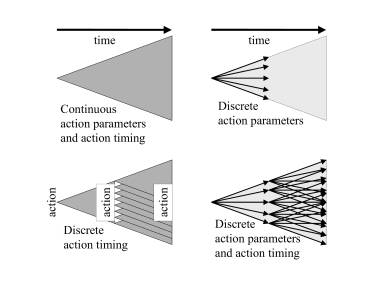Figure 1: Action-based discretization.

Iterative-refinement algorithms use a simple means of dynamically adjusting the time interval between search states. We will present the results of an empirical study of the performance of different search algorithms as one varies the initial time interval between search states. We formalize our generalization of search, describe the algorithms compared, present our chosen class of test problems, and present the experimental results.

## Dynamic Action Timing Discretization

We will assume that the action parameter discretization, i.e. which action parameters are sampled, is already given. From the perspective of the search algorithm, the action discretization is static (cannot be varied by the algorithm). However, action timing discretization is dynamic (can be varied by the algorithm). For this reason, we will call such searches "SADAT searches" as they have Static Action and Dynamic Action Timing discretization.

SADAT searches are different than classical AI searches in only one respect. An action (i.e. operator) additionally takes a time delay parameter indicating how much time will pass before the next action is taken. For dynamical systems where timing is relevant, this is an important generalization.

• State space (a set of possible states).
• An initial state.
• A finite set of actions that map a state and a positive time duration to a successor state and a transition cost.
• Aset of goal states.

In fact, one could view this as having only three parts if you define the state space in terms of the second and third items above for all possible time durations.

In classical search, a goal path can be specified as a sequence of actions that evolve the initial state to a goal state. Now that the timing of actions is a choice, a goal path can be specified as a sequence of action-duration pairs that evolve the initial state to a goal state. The cost of a path is the sum of all transition costs. Given this generalization, the state space is generally infinite, and the optimal path can generally only be approximated through a sampling of possible paths through the state space.

## Algorithms

The following algorithms are all written in Richard Korf’s style of pseudo-code. In an object-oriented implementation, one would naturally have node objects. All of these algorithms simply exit when a goal node is found. Since at any time, the call stack contains all relevant path information for the current node, one could easily modify the algorithms to put node and action information onto a stack while exiting, allowing easy retrieval of the solution path.

## Iterative-Deepening A

All searches have exponential time complexity (O(bd) where b is breadth and d is depth of the search tree.) Depth-first search has linear (O(d)) memory complexity, but does not necessarily find optimal (or even good) solutions. Breadth-first search finds minimum depth solutions, but does so with exponential memory cost. A* search (best-first search with an admissible heuristic) uses a heuristic function to direct search and reduce the cost of search. However, memory complexity is still exponential.

IDA* [Korf, 1985] provides a means of having linear memory complexity and optimality, at the cost of node re-expansion. IDA* performs depth-first searches to successively greater f-value bounds until a solution is found. The pseudo-code for IDA* is as follows:

IDASTAR (node : N)
B := f(N);
WHILE (TRUE)
B := IDASTARB(N, B)

This outermost loop depends on a recursive depth-first search to an f-value bound B:

IDASTARB (node : N, bound : B)
IF N is a goal, EXIT algorithm
IF N has no children, RETURN infinity
FN = infinity
FOR each child Ni of N, F[i] := f(Ni)
IF f(Ni) <= B, FN := MIN(FN, IDASTARB(Ni,B))
ELSE FN := MIN(FN, f(Ni))
RETURN FN

If the recursive search is unsuccessful, it returns the lowest f-value encountered beyond the current bound. The outermost loop then revises the bound to this value and searches to this greater bound. The amount of node re-expansion is problematic if there are many distinct f-values. Such is true of most real-valued search problems. This problem is addressed by the epsilon-admissible variant described below.

Î-Admissible iterative-deepening A* search, here called Î-IDA*, is a version of IDA* where the f-cost limit is increased "by a fixed amount , on each iteration, so that the total number of iterations is proportional to 1/Î. This can reduce the search cost, at the expense of returning solutions that can be worse than optimal by at most Î."[Russell & Norvig, 1995]

Actually, our implementation is an improvement on Î-IDA* as described above. If Df is the difference between (1) the minimum f-value of all nodes beyond the current search contour, and (2) the current f-cost limit, then the f-cost limit is increased by the maximum of Î and Df. (Df is the increase that would occur in IDA*.) Thus, the limit is increased by at least Î or more if the minimum node f-cost beyond the contour exceeds this increase. This improvement is significant in cases where the f-cost limit changes between iterations can significantly exceed Î. The recursive procedure of Î-IDA* is identical to that of IDA*. The difference is in the computation of successive bounds in the outermost loop:

eIDASTAR (node : N)
B := f(N);
WHILE (TRUE)
B := MAX(IDASTARB(N, B), B+Î)

To make this point concrete, suppose the current iteration of Î-IDA* has an f-cost limit of 1.0 and returns no solution and a new f-cost limit of 2.0. The new f-cost limit is the minimum heuristic f-value of all nodes beyond the current search contour. Let us further assume that Î is 0.1. Then increasing the f-cost limit by this fixed Î will result in the useless search of the same contour for 9 more iterations before the new node(s) beyond the contour are searched. In our implementation above, the f-cost limit would instead increase directly to 2.0.

## Recursive Best-First Search

Recursive best-first search (RBFS) [Korf, 1993] is a significant improvement over IDA*. RBFS also expands nodes in best-first order and has linear memory complexity. It also expands fewer nodes than IDA* for nondecreasing cost functions. This is accomplished by some extra bookkeeping concerning node re-expansion. Korf’s RBFS algorithm is as follows:

RBFS (node: N, value: F(N), bound: B)
IF f(N)>B, RETURN f(N)
IF N is a goal, EXIT algorithm
IF N has no children, RETURN infinity
FOR each child Ni of N,
IF f(N) ELSE F[i] := f(Ni)
sort Ni and F[i] in increasing order of F[i]
IF only one child, F := infinity
WHILE (F <= B and F < infinity)
F := RBFS(N1, F, MIN(B, F))
insert Ni and F in sorted order
RETURN F

RBFS suffers from the same problem as IDA* when there are many distinct f-values. This problem is addressed by the new epsilon-admissible variant of RBFS described below.

Î-Admissible recursive best-first search [Neller, 2000], here called Î-RBFS, is a new Î-admissible variant of recursive best-first search [Korf, 1993]. As with our implementation of Î-IDA*, local search bounds increase by at least Î but possibly more as necessary to avoid redundant search.

In Korf's style of pseudo-code, Î-RBFS is as follows:

eRBFS (node: N, value: F(N), bound: B)
IF f(N)>B, RETURN f(N)
IF N is a goal, EXIT algorithm
IF N has no children, RETURN infinity
FOR each child Ni of N,
IF f(N) ELSE F[i] := f(Ni)
sort Ni and F[i] in increasing order of F[i]
IF only one child, F := infinity
WHILE (F <= B and F < infinity)
F := eRBFS(N1, F, MIN(B, MAX(F, F+Î)))
insert Ni and F in sorted order
RETURN F

The difference between RBFS and Î-RBFS is in the computation of the bound for the recursive call. In RBFS, this is computed as MIN(B, F) whereas in Î-RBFS, this is computed as MIN(B, MAX(F, F+Î)). F and F are the lowest and second-lowest stored costs of the children, respectively. Thus, the bound of the recursive call will not exceed that of its parent, and will be the greater of the stored value of the lowest-cost sibling F and its own stored value F plus Î.

The algorithm’s initial call parameters are the root node r, f(r), and ¥. Actually, both RBFS and Î-RBFS can be given a finite bound b if one wishes to restrict search for solutions with a cost of no greater than b, and uses an admissible heuristic function. If no solution is found, the algorithm will return the f-value of the minimum open search node beyond the search contour of b.

In the context of SADAT search problems, both Î-IDA* and Î-RBFS assume a fixed time interval Dt between a node and its child. The following iterative-refinement algorithms do not.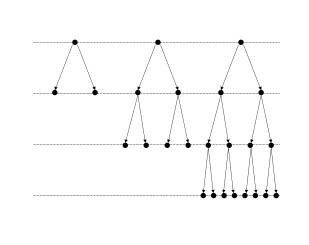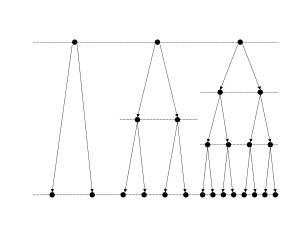Figure 2: Iterative-deepening and iterative-refinement depth-first search.

## Iterative-Refinement

Iterative-refinement [Neller, 2000] is perhaps best described in comparison to iterative-deepening. Iterative-deepening depth-first search (Figure 2(a)) provides both the linear memory complexity benefit of depth-first search and the minimum-length solution-path benefit of breadth-first search at the cost of node re-expansion. Such re-expansion costs are generally dominated by the cost of the final iteration because of the exponential nature of search time complexity.

Iterative-refinement depth-first search (Figure 2(b)) can be likened to an iterative-deepening search to a fixed time-horizon. In classical search problems, time is not an issue. Actions lead from states to other states. When we generalize such problems to include time, we then have the choice of how much time passes between search states. Assuming that the vertical time interval in Figure 2(b) is Dt, we perform successive searches with delays Dt, Dt/2, Dt/3, … until a goal path is found.

Iterative-deepening addresses our lack of knowledge concerning the proper depth of search. Similarly, iterative-refinement addresses our lack of knowledge concerning the proper time discretization of search. Iterative-deepening performs successive searches that grow exponentially in time complexity. The complexity of previous unsuccessful iterations is generally dominated by that of the final successful iteration. The same is true for iterative-refinement.

However, the concept of iterative-refinement is not limited to the use of depth-first search. In general, for each iteration of an iterative-refinement search, a level of (perhaps adaptive) time-discretization granularity is chosen for search and an upper bound on solution cost is given. If the iteration finds a solution within this cost bound, the algorithm terminates with success. Otherwise, a finer level of time-discretization granularity is chosen, and search is repeated. Search is successively refined with respect to time granularity until a solution is found.

## Iterative-Refinement Î-RBFS

Iterative-Refinement Î-RBFS is one instance of such iterative-refinement search. The algorithm can be simply described as follows:

IReRBFS (node: N, bound: B, initDelay: DT)
FOR I = 1 to infinity
Fix the time interval between states at DT/I
eRBFS(N, f(N), B)
IF eRBFS exited with success, EXIT algorithm

Iterative-Refinement Î-RBFS does not search to a fixed time-horizon. Rather, each iteration searches within a search contour bounded by B. Successive iterations search to the same bound, but with finer temporal detail.

## Iterative-Refinement DFS

The algorithm for Iterative-Refinement DFS is given as follows:

IRDFS (node: N, bound: B, initDelay: DT)
FOR I = 1 to infinity
Fix the time interval between states at DT/I
DFS-NOUB(N, f(N), B)
IF DFS-NOUB exited with success, EXIT algorithm

Our depth-first search implementation DFS-NOUB uses a node ordering (NO) heuristic and has a path cost upper bound (UB). The node-ordering heuristic is as usual: Nodes are expanded in increasing order of f-value. Nodes are not expanded that exceed a given cost upper bound. Assuming admissibility of the heuristic function h, no solutions within the cost upper bound will be pruned from search.

Since SADAT search algorithms will generally only be able to approximate optimal solutions, it is helpful to test them on problems with known optimal solutions. Richard Korf proposed the problem of navigation between two points on the surface of a sphere as a simple benchmark with a known optimal solution. Our version of the problem is given here.

The shortest path between two points on a sphere is along the great-circle path. Consider the circle formed by the intersection of a sphere and a plane through two points on the surface of the sphere and the center of the sphere. The great-circle path between the two points is the shorter part of this circle between the two points. The great-circle distance is the length of this path.

Our state space is the set of all positions and headings on the surface of a unit sphere along with all nonnegative time durations for travel. Essentially, we encode path cost (i.e. time) in the state in order to define the goal states. The initial state is arbitrarily chosen to have position (1,0,0) and velocity (0,1,0) in spherical coordinates, with no time elapsed initially.

The action ai, 0<i<7 takes a state and time duration, and returns a new state and the same time duration (i.e. cost = time). The new state is the result of changing the heading i*p/4 radians and traveling with unit velocity at that heading on the surface of the unit sphere. If the position reaches a goal state, the system stops evolving (and incurring cost).

The set of goal states includes all states that are both (1) within Îd great-circle distance from a given position pg, and (2) within ,t time units of the optimal duration to reach such positions. Put differently, the first requirement defines the size and location of the destination, and the second requirement defines how directly the destination must be reached. Position pg is chosen at random from all possible positions on the unit sphere with all positions being equally probable.

If d is the great-circle distance between (1,0,0) and pg, then the optimal time to reach a goal position at unit velocity is d - Îd. Then the solution cost upper bound is d - Îd + Ît.

## Experimental Results

In these experiments, we vary only the initial time delay Dt between search states and observe the performance of the algorithms we have described. For Î-IDA* and Î-RBFS, the initial Dt is the only Dt for search. The iterative-refinement algorithms search using the harmonic refinement sequence Dt, Dt/2, Dt/3, …, and are limited to 1000 refinement iterations.

Experimental results for success rates of search are summarized in Figure 3. Each point represents 500 trials over a fixed, random set of sphere navigation problems with Îd = .0001 and ,t computed as 10 percent of the optimal time. Thus, the target size for each problem is the same, but the varying requirement for solution quality means that different delays will be appropriate for different search problems. Search was terminated after 10 seconds, so the success rate is the fraction of time a solution was found within this allotted time.

In this empirical study, means and 90 percent confidence intervals for the means were computed with 10000 bootstrap resamples.

Let us first compare the performance of iterative-refinement (IR) Î-RBFS and Î-RBFS. To the left of the graph, where the initial Dt0 is small, there is no difference between the two algorithms. This region of the graph indicates conditions under which a solution is found within 10 seconds on the first iteration or not at all. There is no iterative-refinement in this region; the time complexity of the first iteration leaves no time for another.

At about Dt0 = .1, we observe that IR Î-RBFS begins to have a significantly greater success rate than Î-RBFS. At this point, the time complexity of search allows for multiple iterations, and thus we begin to see the benefits of iterative-refinement.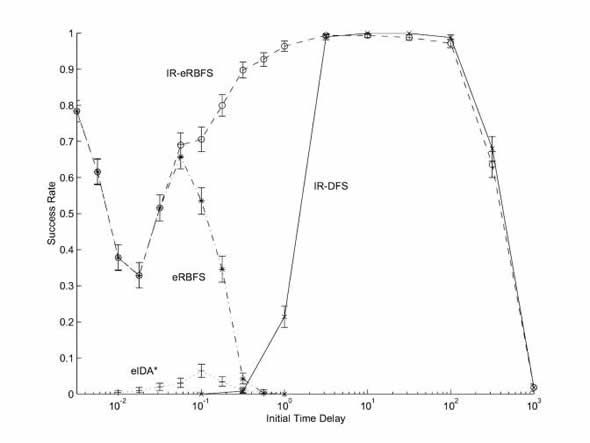Figure 3: Effect of varying initial )t.

Continuing to the right with greater initial Dt0, IR Î-RBFS peaks at a 100 percent success rate. At this point, the distribution of Dt's over different iterations allows IR Î-RBFS to reliably find a solution within the time constraints. We can see the distribution of Dt's that most likely yield solutions from the behavior of Î-RBFS.

Where the success rate of IR Î-RBFS begins to fall, the distribution of first 1000 Dt's begins to fall outside of the region where solutions can be found. With our refinement limit of 1000, the last iteration uses a minimal Dt = Dt0/1000. The highest Dt0 trials fail not because time runs out. Rather, the iteration limit is reached. However, even with a greater refinement limit, we would eventually reach a Dt0 where the iterative search cost incurred on the way to the good Dt range would exceed 10 seconds.

Comparing IR Î-RBFS with IR DFS, we first note that there is little difference between the two for large Dt0. For 3.16<Dt0<100, the two algorithms are almost always able to perform complete searches of the same search contours through all iterations up to the first iteration with a solution path. The largest statistical difference occurs at Dt0 = 316 where IR DFS's success rate is 4.4 percent higher. We note that our implementation of IR DFS has a faster node-expansion rate, and that Î-RBFS's Î-admissibility necessitates significant node re-expansion. For these Dt0's, the use of IR DFS trades off ,-optimality for speed and a slightly higher success rate.

For low-to-mid-range Dt0 values, however, we begin to see the efficiency of Î-RBFS over DFS with node ordering as the first iteration with a solution path presents a more computationally costly search. Since the target destination is so small, the route that actually leads through the target destination is not necessarily the most direct route. Without a perfect heuristic where complex search is necessary, Î-RBFS shows its strength relative to DFS. Rarely will problems be so unconstrained and offer such an easyheuristic as this benchmark problem, so IR Î-RBFS will be generally be better suited for all but the simplest search problems.

Comparing IR Î-RBFS with Î-IDA*, we note that Î-IDA* performs relatively poorly over all Dt0. What is particularly interesting is the performance of Î-IDA* over the range where IR Î-RBFS behaves as Î-RBFS, i.e. where no iterative-refinement takes place. Here we have empirical confirmation of the significant efficiency of Î-RBFS over Î-IDA*.

In summary, iterative-refinement algorithms are statistically the same as or superior the other searches over the range of Dt0 values tested. IR Î-RBFS offers the greatest average success rate across all Dt0. With respect to Î-RBFS, IR Î-RBFS offers significantly better performance for Dt0 spanning more than four orders of magnitude. These findings are in agreement with previous empirical studies concerning a submarine detection avoidance problem [Neller, 2000].

This is significant for search problems where reasonable values for Dt are unknown. This is also significant for search problems where reasonable values for Dt are known and one wishes to find a solution more quickly and reliably. This performance comes at a reasonable price for many applications. Lack of knowledge of a good time discretization is compensated for by knowledge of a suitable solution cost upper bound.

## Dynamic Action Parameter Discretization

Having looked at some methods for performing dynamic action timing discretization, we will now focus on dynamic action parameter discretization. Now we will assume that the action timing discretization, i.e. when actions are taken, is already given. From the perspective of the search algorithm, the action timing discretization is static (cannot be varied by the algorithm). However, the action parameter discretization is dynamic (can be varied by the algorithm). For this reason, we will call such searches "DASAT searches" as they have Dynamic Action and Static Action Timing discretization.

DASAT are different than classical AI searches in only one respect. There are infinite ranges of action parameters. In the context of navigation, the choice of a heading change can come from an infinite continuum of angle choices 0 - 2p. For dynamical systems where the choice of action parameters is relevant, this is an important generalization.

A DASAT search problem is made up of four parts:

• State space (a set of possible states).
• An initial state.
• Afinite set of actions regions which define possible parameter ranges for actions, where each point in the region represents an action that maps a state to a successor state and a transition cost.
• Aset of goal states.

Given this generalization, the state space is generally infinite, and the optimal path can generally only be approximated through a sampling of possible paths through the state space.

When both action parameter and action timing discretizations are dynamic, we call such searches “DADAT” searches. The following experiments were performed with DADAT searches using a form of iterative-refinement depth-first search and three different forms of action parameter discretization.

## Submarine Channel Problem

The Submarine Channel Problem is not unlike a Sega videogame of the 1980's called Frogger. A submarine seeks a path through a channel such that it avoids being detected by a number of patrolling ships. We have chosen this problem because, like the n2-1 sliding tile puzzles, it can serve as a benchmark easily scalable to greater difficulty.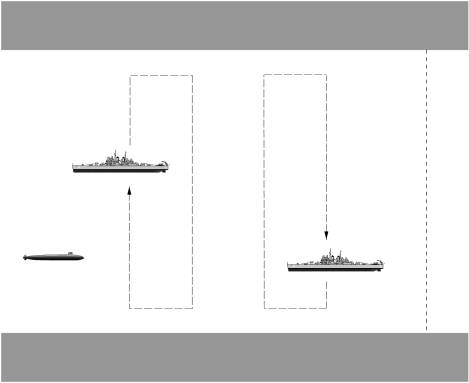Figure 4: Submarine Channel Problem

In the Submarine Channel Problem, the submarine starts at position (x, y) = (0, 0) with eastward heading and at full stop. To the east along an east-west channel of width w (centered along y=0) are n ships patrolling across the width of the channel. This is pictured in Figure 4.

Each ship j has an inner detection radius ri,j and an outer detection radius ro,j. Within a proximity of ri,j, ship j will detect the submarine and the submarine will be penalized with a detection penalty. Within a proximity of ro,j and beyond ri,j, the submarine incurs a proximity penalty scaling linearly from 0 at the outer radius to the full detection penalty at the inner radius. Beyond the outer radius, there is no penalty. If the submarine collides with the sides of the channel, there is a collision penalty. In the case of collision or detection, the submarine is halted and allowed no further legal moves. The first ship patrols at an x-offset xOffset1 = ro,1. Each ship k thereafter has xOffsetk = xOffsetk-1 + 3ri,k-1 + ri,k. Ship k has a patrolling route defined by cycling linearly between the following points: (xOffsetk, w/2-ri,k), (xOffsetk+2ri,k, w/2-ri,k ), (xOffsetk +2ri,k, -w/2+ri,k), and (xOffsetk, -w/2 + ri,k). Each ship begins at a given percentage along this cycle. For n ships, the goal states are all states within the channel with x > xOffsetn + 2ri,n + ro,n, i.e. all channel points to the right of the rightmost outer detection radius.

The submarine can travel in eight headings (multiples of p/4 radians), and three speeds: full speed, half speed, and full stop. Together these define 17 distinct actions the submarine can take at any point which it has incurred neither collision nor full detection penalty. (Since we assume discrete, instantaneous changes to headings and speeds, all full stop actions are effectively equivalent.) Each ship travels at a single predefined speed.

## Random, Uniform, and Dispersed Discretization

Generalizing the submarine channel problem for DADAT search, we allow any heading and any speed up to the maximum. Thus an action, i.e. changing heading and speed, can be thought of as picking a point in a circular region with the radius being the maximum speed. The center point is a full stop, and any other point indicates a heading and speed (in polar coordinates).

Faced with this freedom of choice in our search algorithms, we present three ways of performing dynamic action parameter discretization. First, we can randomly choose parameters with independent uniform distributions over headings and speeds. Second, we can take a fixed uniform discretization as described above and rotate it by a random angle. Third, we can seek to generate a discretization with action parameters as “far” from each other as possible. We call this last technique “dispersed discretization”.

The basic idea of “dispersed” discretization is to take a number of randomly sampled points from the action region and simulate them as if they were point charges mutually repelling each other with force proportional to the inverse square of their distance. The point dispersion algorithm pseudo-code is as follows:

DISPERSE (REGION, SAMPLES, WEIGHT, DECAY, ITERATIONS)
FOR I = 1 to SAMPLES
X[I] := random point in REGION
FOR I = 1 to ITERATIONS
FOR J = 1 to SAMPLES
DX[J] := 0
FOR K = 1 to J
DIFFERENCE := X[K] – X[J]
DISTANCE := SQRT(X[J]2 + X[K]2)
DX[J] := DX[J] – DIFFERENCE/(DISTANCE3)
DX[K] := DX[K] + DIFFERENCE/(DISTANCE3)
FOR J = 1 to SAMPLES
DX[J] := WEIGHT * DX[J]
X[J] := X[J] + DX[J]
IF X[J] not in REGION,
reassign X[J] to the closest point in the REGION
WEIGHT := WEIGHT * DECAY
RETURN X

We used a repulsion factor of 0.008 and a repulsion factor decay of 0.93 for 20 iterations. These values were chosen empirically based on a small number of trials with the submarine action region. In future work, we would desire these dispersion parameters to be rapidly self-adapting to the size of the region and the number of sampled points.

## Experimental Results

For these experiments, we have chosen w=1 length unit. The outer radius of each ship is 0.2w. The inner radius of each ship is 0.1w. The maximum velocity of the submarine is w/(1 time unit). All ship velocities are also w/(1 time unit). Ships are started at random percentages through their patrol cycles. The detection and collision penalties are set at 10000. In each experimental trial we generated a random 10-ship submarine channel problem. A successful trial found a solution within 10 seconds of search. For each initial time delay Dt we ran 100 trials.

Figure 5 summarizes experimental results comparing the performance of random, uniform, and dispersed discretization techniques used with a form of iterative-refinement depth-first search. Note that the dispersed discretization rate of success exceeds that of the other discretization techniques.

Looking over a number of dispersed discretizations, one quickly notices that more points are repelled to the edge than in the uniform discretization. Although not a probable configuration, any number of points placed at even intervals around the edge would be in equilibrium. With repulsion parameters given above, it was typical to see 12 or more points along the edge of the circle with five or fewer points dispersed internally. Empirically, extreme parameters represented by the edge of the circular action region are more likely to appear in optimal solutions. We hypothesize that having extra edge action choices aids in finding better approximations to optimal solutions.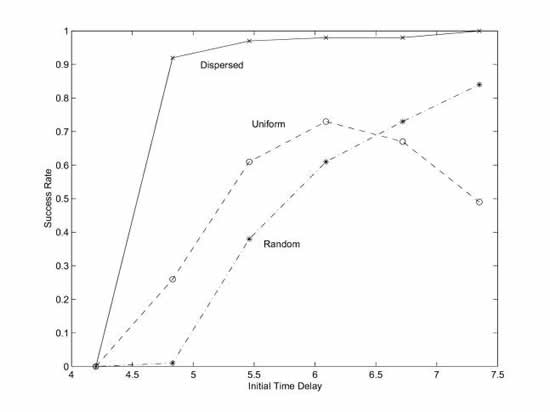Figure 5: Varying initial delay with different action parameter discretizations.

Furthermore, in this problem domain, searches of faster submarine trajectories (i.e. with discretizations having more maximal velocities) will have lesser search depths to solutions if such speedy solution trajectories exist. Since search depth affects search time complexity exponentially, we likely benefit from a discretization with more maximal velocity values.

One key lesson in this and other experiments of [Neller, 2000] is that behaviors of greatest interest often occur at extreme parameter values. Another key lesson is that an automated discretization technique outperformed one hand-chosen by researchers (uniform). Not only can such discretization techniques reduce the discretization burden of the programmer; they may also yield superior discretizations.

## Conclusions

Artificial Intelligence search algorithms search discrete systems. To apply such algorithms to continuous systems, such systems must first be discretized, i.e. approximated as discrete systems. Action-based discretization requires that both action parameters and action timing be discretized.

The empirical study concerning sphere navigation provided insight into the importance of searching with dynamic time discretization. Iterative-refinement algorithms are given an initial time delay Dt0 between search states and a solution cost upper bound. Such algorithms iteratively search to this bound with successively smaller Dt until a solution is found.

Iterative-refinement ,-admissible recursive best-first search (IR Î-RBFS) was shown to be similar or superior to all other searches studied for Dt0 spanning over five orders of magnitude. With respect to Î-RBFS (without iterative-refinement), a new ,-admissible variant of Korf's recursive best-first search, IR Î-RBFS offers significantly better performance for Dt0 spanning over four orders of magnitude.

Iterative-refinement algorithms are important for search problems where reasonable values for Dt are unknown or known and one wishes to find a solution more quickly and reliably. The key tradeoff is that of knowledge. Lack of knowledge of a good time discretization is compensated for by knowledge of a suitable solution cost upper bound. If one knows a suitable solution cost upper bound for a problem where continuous time is relevant, an iterative-refinement algorithm such as IR Î-RBFS is recommended.

Finally, in the context of a submarine detection avoidance problem, we introduced a dispersed discretization technique for dynamically choosing action parameters. The resulting discretization was superior to a uniform discretization chosen by researchers.
Here we have presented a few techniques for dynamically discretizing both action parameters and action timings of continuous search problems with empirical evidence of their benefits. We see this work as providing first steps in a class of algorithms that will prove important in the application of AI search algorithms to continuous problem domains.

## Bibliography

Korf, R. E. 1985. Depth-first iterative-deepening: an optimal admissible tree search. Artificial Intelligence 27(1):97-109.

Korf, R. E. 1993. Linear-space best-first search. Artificial Intelligence 62:41-78.

Mataric, M. J. 2000. Sensory-motor primitives as a basis for imitation: Linking perception
to action and biology to robotics. In Nehaniv, C., and Dautenhahn, K., eds., Imitation in Animals and Artifacts. Cambridge, MA, USA: MIT Press. See also USC technical report IRIS-99-377.

Neller, T. W. 2000. Simulation-Based Search for Hybrid System Control and Analysis, Ph.D. Dissertation, Stanford University, Palo Alto, CA, USA. Also available as Stanford Knowledge Systems Laboratory technical report KSL-00-15 at www.ksl.stanford.edu.

Russell, S., and Norvig, P. 1995. Artificial Intelligence: a Modern Approach. Upper Saddle River, NJ, USA: Prentice Hall.

______________________________________________________

### Latest Jobs

#### Treyarch

Playa Vista, Los Angeles, CA, USA
2.03.23
Senior Physics Gameplay Engineer - Treyarch

#### High Moon Studios

2.03.23
VFX Artist - High Moon Studios

#### Anne Arundel Community College

Arnold, MD, USA
1.30.23
Instructor/Assistant Professor, Game Art

#### Treyarch Vancouver

2.02.23
Producer - Treyarch Vancouver
More Jobs

#### Game Developer Job Board

Browse open positions across the game industry or recruit new talent for your studio